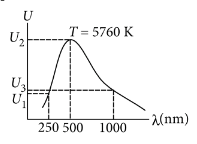Question

# A black body is at a temperature of . The energy of radiation emitted by the body at wavelength  is${U}_{1}$ , at wavelength  is ${U}_{2}$ and that at  is ${U}_{3}$. Wien’s constant, $b=2.88×{10}^{6}nmK$. Which of the following is correct?

Moderate
Solution

## According to Wein's displacement law${U}_{1}<{U}_{2}>{U}_{3}$

Get Instant Solutions